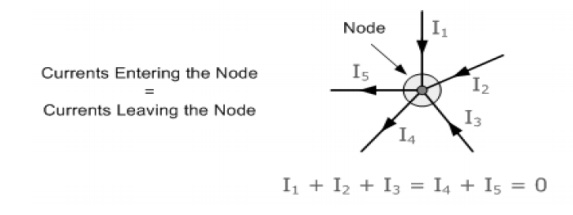Home | | Circuit Theory | Kirchoff's Law with Example Problems and Calculations

# Kirchoff's Law with Example Problems and Calculations

Kirchoff's First Law - The Current Law, (KCL), Kirchoff's Second Law - The Voltage Law, (KVL)

KIRCHOFF'S LAW

Kirchoff's First Law - The Current Law, (KCL)

"The total current or charge entering a junction or node is exactly equal to the charge leaving the node as it has no other place to go except to leave, as no charge is lost within the node".

In other words the algebraic sum of ALL the currents entering and leaving a node must be equal to zero,

I(exiting) + I(entering) = 0.

This idea by Kirchoff is known as the Conservation of Charge.Here, the 3 currents entering the node, I1, I2, I3 are all positive in value and the 2 currents leaving the node, I4 and I5 are negative in value.

Then this means we can also rewrite the equation as; I1 + I2 + I3 - I4 - I5 = 0

Kirchoff's Second Law - The Voltage Law, (KVL)

"In any closed loop network, the total voltage around the loop is equal to the sum of all the voltage drops within the same loop" which is also equal to zero. In other words the algebraic sum of all voltages within the loop must be equal to zero. This idea by Kirchoff is known as the Conservation of Energy.

Starting at any point in the loop continue in the same direction noting the direction of all the voltage drops, either positive or negative, and returning back to the same starting point. It is important to maintain the same direction either clockwise or anti-clockwise or the final voltage sum will not be equal to zero.

We can use Kirchoff's voltage law when analyzing series circuits.Problem 1:

A current of 0.5 A is flowing through the resistance of 10â„¦.Findthepotential difference between its ends.

Solution:

Current I     = 0.5A.

Resistance R = 10â„¦

Potential difference V =?

V = IR

=   0.5 Ă— 10

=   5V.

Problem: 2

A supply voltage of 220V is applied to a resistor100.Findâ„¦the current flowing through it.

Solution:

Voltage V = 220V Resistance R = 100â„¦ Current I = V/ R

=   2 2 0 /100

=   2.2 A.

Problem: 3

Calculate the resistance of the conductor if a current of 2A flows through it when the potential difference across its ends is 6V.

Solution:

Current I = 2A. Potential difference = V = 6. Resistance R = V/I

=   6 /2

=   3 ohm.

Problem: 4

Calculate the current and resistance of a 100 W, 200V electric bulb.

Solution:

Power, P = 100W

Voltage, V = 200V Power p = VI

Current I = P/V

=   100/200

=     0.5A

Resistance R = V /I

= 200/0.5

= 400W.

Problem: 5

Calculate the power rating of the heater coil when used on 220V supply taking 5 Amps.

Solution:

Voltage, V = 220V Current, I = 5A, Power, P = VI

=   220 Ă— 5

=   1100W

=   1.1 KW.

Problem: 6

A circuit is made of 0.4wire,â„¦a 150bulbâ„¦and a rheostat120connectedâ„¦ in series. Determine the total resistance of the resistance of the circuit.

Solution:

Resistance of the wire = 0.4Resistanceâ„¦ of bulb  = 1 5 0 â„¦ Resistance of rheostat =  120â„¦

In series,

Total resistance, R = 0.4 + 150 +120 = 270.4â„¦

Problem : 7

Three  resistances  of  values   2â„¦,3â„¦connectedandin  series5â„¦  acrossare20  V,D.C  supply

.Calculate (a) equivalent resistance of the circuit (b) the total current of the circuit (c) the voltage drop across each resistor and (d) the power dissipated in each resistor.

Solution:

Total resistance R  = R1 + R 2+ R3.

=   2   +3+5   =   10â„¦

Voltage       = 20V

Total current  I     = V/R = 20/10  = 2A.

Voltage drop across 2â„¦resistor V1  =       I R1

= 2Ă— 2  = 4 volts.

Voltage drop across 3â„¦resistor V 2 = IR2

= 2 Ă— 3 = 6 volts.

Voltage drop across 5â„¦resistor        V3 = I R3

= 2 Ă—5 = 10 volts.

Power dissipated in 2â„¦resistor is P1 = I2 R1

= 22 Ă— 2  = 8 watts.

Power dissipated in 3 resistor is P2            = I2 R2.

= 22 Ă— 3 = 12 watts.

Power dissipated in 5 resistor is P3            = I2 R3

= 22 Ă— 5 = 20 watts.

Problem: 8

A lamp can work on a 50 volt mains taking 2 amps. What value of the resistance must be connected in series with it so that it can be operated from 200 volt mains giving the same power.

Solution:

Lamp voltage, V =  50V Current, I = 2 amps.

Resistance of the lamp = V/I = 50/25   =   25   â„¦

Resistance connected in series with lamp = r.

Supply voltage = 200 volt.

Circuit current I = 2A

Total resistance Rt= V/I   =   200/2   =   100â„¦

Rt = R  + r 100 = 25 + r

r =   75â„¦

Problem: 9

Find  the   current   flowing   in   the   40Î©   Resistor,Solution:

The circuit has 3 branches, 2 nodes (A and B) and 2 independent loops.

Using Kirchoff's Current Law, KCL the equations are given as;

At node A: I1 + I2 = I3

At node B: I3 = I1 + I2

Using Kirchoff's Voltage Law, KVL the equations are given as;

Loop 1 is given as: 10 = R1 x I1 + R3 x I3 = 10I1 + 40I3

Loop 2 is given as: 20 = R2 x I2 + R3 x I3 = 20I2 + 40I3

Loop 3 is given as: 10 - 20 = 10I1 - 20I2

As I3 is the sum of I1 + I2 we can rewrite the equations as;

Eq. No 1: 10 = 10I1 + 40(I1 + I2) = 50I1 + 40I2

Eq.No 2: 20 = 20I1 + 40(I1 + I2) = 40I1 + 60I2

We now have two "Simultaneous Equations" that can be reduced to give us the value of both I1 and

I2

Substitution of I1 in terms of I2 gives us the value of I1 as -0.143 Amps

Substitution of I2 in terms of I1 gives us the value of I2 as +0.429 Amps

As: I3 = I1 + I2

The current flowing in resistor R3 is given as: -0.143 + 0.429 = 0.286 Amps and the voltage across the resistor R3 is given as : 0.286 x 40 = 11.44 volts

Problem: 10

Find the current in a circuit using Kirchhoff's voltage lawStudy Material, Lecturing Notes, Assignment, Reference, Wiki description explanation, brief detail
Electrical and electronics : Circuit Theory : Kirchoff's Law with Example Problems and Calculations |